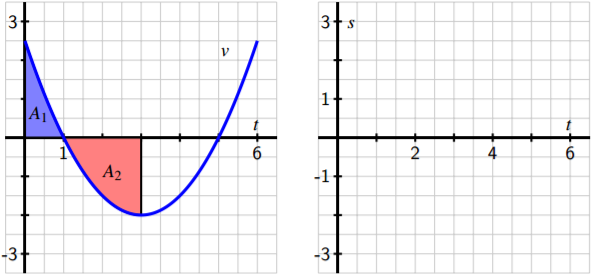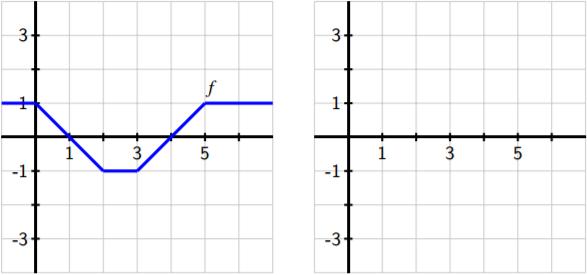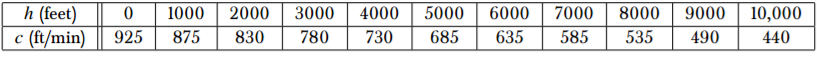$$\newcommand{\id}{\mathrm{id}}$$ $$\newcommand{\Span}{\mathrm{span}}$$ $$\newcommand{\kernel}{\mathrm{null}\,}$$ $$\newcommand{\range}{\mathrm{range}\,}$$ $$\newcommand{\RealPart}{\mathrm{Re}}$$ $$\newcommand{\ImaginaryPart}{\mathrm{Im}}$$ $$\newcommand{\Argument}{\mathrm{Arg}}$$ $$\newcommand{\norm}{\| #1 \|}$$ $$\newcommand{\inner}{\langle #1, #2 \rangle}$$ $$\newcommand{\Span}{\mathrm{span}}$$

5.E: Finding Antiderivatives and Evaluating Integrals (Exercises)

$$\newcommand{\vecs}{\overset { \rightharpoonup} {\mathbf{#1}} }$$ $$\newcommand{\vecd}{\overset{-\!-\!\rightharpoonup}{\vphantom{a}\smash {#1}}}$$$$\newcommand{\id}{\mathrm{id}}$$ $$\newcommand{\Span}{\mathrm{span}}$$ $$\newcommand{\kernel}{\mathrm{null}\,}$$ $$\newcommand{\range}{\mathrm{range}\,}$$ $$\newcommand{\RealPart}{\mathrm{Re}}$$ $$\newcommand{\ImaginaryPart}{\mathrm{Im}}$$ $$\newcommand{\Argument}{\mathrm{Arg}}$$ $$\newcommand{\norm}{\| #1 \|}$$ $$\newcommand{\inner}{\langle #1, #2 \rangle}$$ $$\newcommand{\Span}{\mathrm{span}}$$ $$\newcommand{\id}{\mathrm{id}}$$ $$\newcommand{\Span}{\mathrm{span}}$$ $$\newcommand{\kernel}{\mathrm{null}\,}$$ $$\newcommand{\range}{\mathrm{range}\,}$$ $$\newcommand{\RealPart}{\mathrm{Re}}$$ $$\newcommand{\ImaginaryPart}{\mathrm{Im}}$$ $$\newcommand{\Argument}{\mathrm{Arg}}$$ $$\newcommand{\norm}{\| #1 \|}$$ $$\newcommand{\inner}{\langle #1, #2 \rangle}$$ $$\newcommand{\Span}{\mathrm{span}}$$

5.1: Construction Accurate Graphs of Antiderivatives

1. A moving particle has its velocity given by the quadratic function v pictured in Figure 5.6. In addition, it is given that A1 = 7 6 and A2 = 8 3 , as well as that for the corresponding position function s, s(0) = 0.5.

(a) Use the given information to determine s(1), s(3), s(5), and s(6).

(b) On what interval(s) is s increasing? On what interval(s) is s decreasing?

(c) On what interval(s) is s concave up? On what interval(s) is s concave down?Figure 5.6: At left, the given graph of v. At right, axes for plotting s.

(d) Sketch an accurate, labeled graph of s on the axes at right in Figure 5.6.

(e) Note that v(t) = −2 + 1 2 (t − 3) 2 . Find a formula for s.

2. A person exercising on a treadmill experiences different levels of resistance and thus burns calories at different rates, depending on the treadmill’s setting. In a particular workout, the rate at which a person is burning calories is given by the piecewise constant function c pictured in Figure 5.7. Note that the units on c are “calories per minute.”Figure 5.7: At left, the given graph of c. At right, axes for plotting C.

(a) Let C be an antiderivative of c. What does the function C measure? What are its units?

(b) Assume that C(0) = 0. Determine the exact value of C(t) at the values t = 5, 10, 15, 20, 25, 30. 5.1. CONSTRUCTING ACCURATE GRAPHS OF ANTIDERIVATIVES 275

(c) Sketch an accurate graph of C on the axes provided at right in Figure 5.7. Be certain to label the scale on the vertical axis.

(d) Determine a formula for C that does not involve an integral and is valid for 5 ≤ t ≤ 10.

3. Consider the piecewise linear function f given in Figure 5.8. Let the functions A, B, and C be defined by the rules A(x) = R x −1 f (t) dt, B(x) = R x 0 f (t) dt, and C(x) = R x 1 f (t) dt.Figure 5.8: At left, the given graph of f . At right, axes for plotting A, B, and C.

(a) For the values x = −1, 0, 1, . . ., 6, make a table that lists corresponding values of A(x), B(x), and C(x).

(b) On the axes provided in Figure 5.8, sketch the graphs of A, B, and C.

(c) How are the graphs of A, B, and C related?

(d) How would you best describe the relationship between the function A and the function f?

5.2: The Second Fundamental Theorem of Calculus

1. Let g be the function pictured at left in Figure 5.13, and let F be defined by F(x) = R x 2 g(t) dt. Assume that the shaded areas have values A1 = 4.29, A2 = 12.75, A3 = 0.36, and A4 = 1.79. Assume further that the portion of A2 that lies between x = 0.5 and x = 2 is 6.06. Sketch a carefully labeled graph of F on the axes provided, and include a written analysis of how you know where F is zero, increasing, decreasing, CCU, and CCD. 2. The tide removes sand from the beach at a small ocean park at a rate modeled by the function R(t) = 2 + 5 sin 4πt 25 A pumping station adds sand to the beach at rate modeled by the function S(t) = 15t 1 + 3t Both R(t) and S(t) are measured in cubic yards of sand per hour, t is measured in hours, and the valid times are 0 ≤ t ≤ 6. At time t = 0, the beach holds 2500 cubic yards of sand.Figure 5.13: At left, the graph of g. At right, axes for plotting F.

(a) What definite integral measures how much sand the tide will remove during the time period 0 ≤ t ≤ 6? Why?

(b) Write an expression for Y(x), the total number of cubic yards of sand on the beach at time x. Carefully explain your thinking and reasoning.

(c) At what instantaneous rate is the total number of cubic yards of sand on the beach at time t = 4 changing?

(d) Over the time interval 0 ≤ t ≤ 6, at what time t is the amount of sand on the beach least? What is this minimum value? Explain and justify your answers fully.

3. When an aircraft attempts to climb as rapidly as possible, its climb rate (in feet per minute) decreases as altitude increases, because the air is less dense at higher altitudes. Given below is a table showing performance data for a certain single engine aircraft, giving its climb rate at various altitudes, where c(h) denotes the climb rate of the airplane at an altitude h.Let a new function m, that also depends on h, (say y = m(h)) measure the number of minutes required for a plane at altitude h to climb the next foot of altitude.

a. Determine a similar table of values for m(h) and explain how it is related to the table above. Be sure to discuss the units on m.

b. Give a careful interpretation of a function whose derivative is m(h). Describe what the input is and what the output is. Also, explain in plain English what the function tells us. 5.2. THE SECOND FUNDAMENTAL THEOREM OF CALCULUS 287

c. Determine a definite integral whose value tells us exactly the number of minutes required for the airplane to ascend to 10,000 feet of altitude. Clearly explain why the value of this integral has the required meaning.

d. Determine a formula for a function M(h) whose value tells us the exact number of minutes required for the airplane to ascend to h feet of altitude.

e. Estimate the values of M(6000) and M(10000) as accurately as you can. Include units on your results.

5.3 Integration by Substitution

Exercises

1. This problem centers on finding antiderivatives for the basic trigonometric functions other than sin(x) and cos(x).

(a) Consider the indefinite integral Z tan(x) dx. By rewriting the integrand as tan(x) = sin(x) cos(x) and identifying an appropriate function-derivative pair, make a u-substitution and hence evaluate Z tan(x) dx.

(b) In a similar way, evaluate Z cot(x) dx.

(c) Consider the indefinite integral Z sec2 (x) + sec(x)tan(x) sec(x) + tan(x) dx. Evaluate this integral using the substitution u = sec(x) + tan(x).

(d) Simplify the integrand in (c) by factoring the numerator. What is a far simpler way to write the integrand?

(e) Combine your work in (c) and (d) to determine R sec(x) dx. (f) Using (c)-(e) as a guide, evaluate Z csc(x) dx.

2. Consider the indefinite integral Z x √ x − 1 dx.

(a) At first glance, this integrand may not seem suited to substitution due to the presence of x in separate locations in the integrand. Nonetheless, using the composite function √ x − 1 as a guide, let u = x − 1. Determine expressions for both x and dx in terms of u.

(b) Convert the given integral in x to a new integral in u.

(c) Evaluate the integral in (b) by noting that √ u = u 1/2 and observing that it is now possible to rewrite the integrand in u by expanding through multiplication.

(d) Evaluate each of the integrals Z x 2 √ x − 1 dx and Z x √ x 2 − 1 dx. Write a 298 5.3. INTEGRATION BY SUBSTITUTION paragraph to discuss the similarities among the three indefinite integrals in this problem and the role of substitution and algebraic rearrangement in each.

3. Consider the indefinite integral Z sin3 (x) dx.

(a) Explain why the substitution u = sin(x) will not work to help evaluate the given integral.

(b) Recall the Fundamental Trigonometric Identity, which states that sin2 (x) + cos2 (x) = 1. By observing that sin3 (x) = sin(x) · sin2 (x), use the Fundamental Trigonometric Identity to rewrite the integrand as the product of sin(x) with another function.

(c) Explain why the substitution u = cos(x) now provides a possible way to evaluate the integral in (b).

(d) Use your work in (a)-(c) to evaluate the indefinite integral Z sin3 (x) dx.

(e) Use a similar approach to evaluate Z cos3 (x) dx.

4. For the town of Mathland, MI, residential power consumption has shown certain trends over recent years. Based on data reflecting average usage, engineers at the power company have modeled the town’s rate of energy consumption by the function r(t) = 4 + sin(0.263t + 4.7) + cos(0.526t + 9.4). Here, t measures time in hours after midnight on a typical weekday, and r is the rate of consumption in megawatts5 at time t. Units are critical throughout this problem.

(a) Sketch a carefully labeled graph of r(t) on the interval [0,24] and explain its meaning. Why is this a reasonable model of power consumption?

(b) Without calculating its value, explain the meaning of R 24 0 r(t) dt. Include appropriate units on your answer.

(c) Determine the exact amount of power Mathland consumes in a typical day.

(d) What is Mathland’s average rate of energy consumption in a given 24-hour period? What are the units on this quantity? 5The unit megawatt is itself a rate, which measures energy consumption per unit time. A megawatt-hour is the total amount of energy that is equivalent to a constant stream of 1 megawatt of power being sustained for 1 hour.

5.4: Integration by Parts

1. Let f (t) = te−2t and F(x) = R x 0 f (t) dt.

(a) Determine F 0 (x).

(b) Use the First FTC to find a formula for F that does not involve an integral.

(c) Is F an increasing or decreasing function for x > 0? Why?

2. Consider the indefinite integral given by R e 2x cos(e x ) dx.

(a) Noting that e 2x = e x · e x , use the substitution z = e x to determine a new, equivalent integral in the variable z.

(b) Evaluate the integral you found in (a) using an appropriate technique.

(c) How is the problem of evaluating R e 2x cos(e 2x ) dx different from evaluating the integral in (a)? Do so.

(d) Evaluate each of the following integrals as well, keeping in mind the approach(es) used earlier in this problem:

• R e 2x sin(e x ) dx

• R e 3x sin(e 3x ) dx

• R xex 2 cos(e x 2 )sin(e x 2 ) dx

3. For each of the following indefinite integrals, determine whether you would use usubstitution, integration by parts, neither*, or both to evaluate the integral. In each case, write one sentence to explain your reasoning, and include a statement of any substitutions used. (That is, if you decide in a problem to let u = e 3x , you should state that, as well as that du = 3e 3x dx.) Finally, use your chosen approach to evaluate each integral. (* one of the following problems does not have an elementary antiderivative and you are not expected to actually evaluate this integral; this will correspond with a choice of “neither” among those given.)

(a) R x 2 cos(x 3 ) dx

(b) R x 5 cos(x 3 ) dx (Hint: x 5 = x 2 · x 3 )

(c) R x ln(x 2 ) dx

(d) R sin(x 4 ) dx

(e) R x 3 sin(x 4 ) dx

(f) R x 7 sin(x 4 ) dx

5.5: Other Options for Finding Algebraic Derivatives

1. For each of the following integrals involving rational functions, (1) use a CAS to find the partial fraction decomposition of the integrand; (2) evaluate the integral of the resulting function without the assistance of technology; (3) use a CAS to evaluate the original integral to test and compare your result in (2).

(a) Z x 3 + x + 1 x 4 − 1 dx

(b) Z x 5 + x 2 + 3 x 3 − 6x 2 + 11x − 6 dx 318 5.5. OTHER OPTIONS FOR FINDING ALGEBRAIC ANTIDERIVATIVES

(c) Z x 2 − x − 1 (x − 3) 3 dx

2. For each of the following integrals involving radical functions, (1) use an appropriate u-substitution along with Appendix A to evaluate the integral without the assistance of technology, and (2) use a CAS to evaluate the original integral to test and compare your result in (1).

(a) Z 1 x √ 9x 2 + 25 dx

(b) Z x √ 1 + x 4 dx

(c) Z e x √ 4 + e 2x dx

(d) Z tan(x) p 9 − cos2(x) dx

3. Consider the indefinite integral given by Z q x + √ 1 + x 2 x dx.

(a) Explain why u-substitution does not offer a way to simplify this integral by discussing at least two different options you might try for u.

(b) Explain why integration by parts does not seem to be a reasonable way to proceed, either, by considering one option for u and dv.

(c) Is there any line in the integral table in Appendix A that is helpful for this integral?

(d) Evaluate the given integral using WolframAlpha. What do you observe?

5.6: Numerical Integration

1. Consider the definite integral R 1 0 x tan(x) dx.

(a) Explain why this integral cannot be evaluated exactly by using either usubstitution or by integrating by parts.

(b) Using 4 subintervals, compute L4, R4, M4, T4, and S4.

(c) Which of the approximations in (b) is an over-estimate to the true value of R 1 0 x tan(x) dx? Which is an under-estimate? How do you know?

2. For an unknown function f (x), the following information is known.

• f is continuous on [3, 6];

• f is either always increasing or always decreasing on [3, 6];

• f has the same concavity throughout the interval [3, 6];

• As approximations to R 6 3 f (x) dx, L4 = 7.23, R4 = 6.75, and M4 = 7.05. 11

One reason that Simpson’s Rule is so effective is that S2n benefits from using 2n + 1 points of data. Because it combines Mn, which uses n midpoints, and Tn, which uses the n + 1 endpoints of the chosen subintervals, S2n takes advantage of the maximum amount of information we have when we know function values at the endpoints and midpoints of n subintervals. 5.6. NUMERICAL INTEGRATION 331

(a) Is f increasing or decreasing on [3, 6]? What data tells you?

(b) Is f concave up or concave down on [3, 6]? Why?

(c) Determine the best possible estimate you can for R 6 3 f (x) dx, based on the given information.

3. The rate at which water flows through Table Rock Dam on the White River in Branson, MO, is measured in thousands of cubic feet per second (TCFS). As engineers open the floodgates, flow rates are recorded according to the following chart.(a) What definite integral measures the total volume of water to flow through the dam in the 60 second time period provided by the table above?

(b) Use the given data to calculate Mn for the largest possible value of n to approximate the integral you stated in (a). Do you think Mn over- or underestimates the exact value of the integral? Why?

(c) Approximate the integral stated in (a) by calculating Sn for the largest possible value of n, based on the given data.

(d) Compute 1 60 Sn and 2000+2100+2400+3000+3900+5100+6500 7 . What quantity do both of these values estimate? Which is a more accurate approximation?